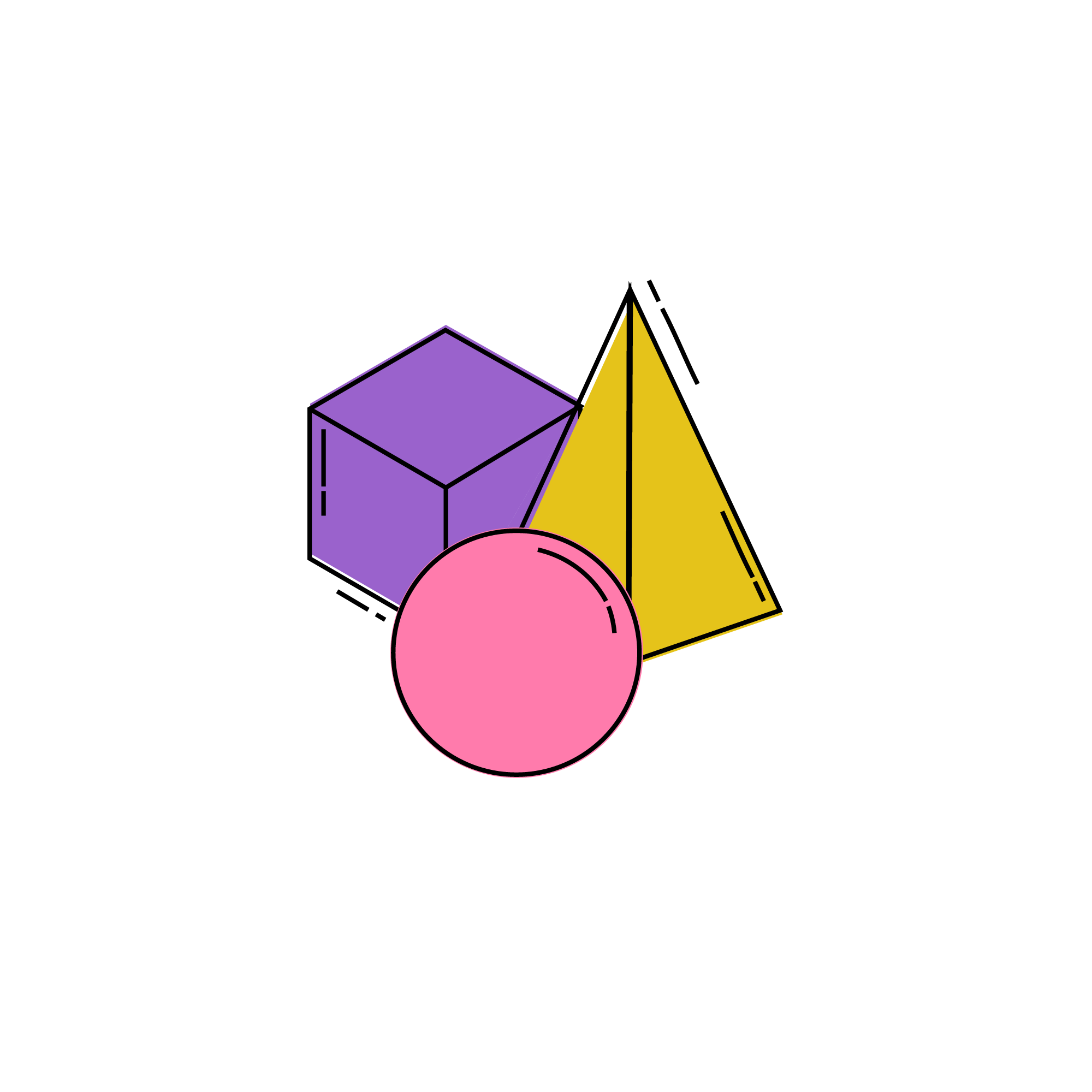ACT Math Geometry

## 1. Cubes

#### A CUBE is created by the connection of six square faces. The formula for the volume of a cube is as follows. Volume is a representation of the amount of space that the shape takes up. You can also think of it as the amount of liquid that could fill the three-dimensional shape.

$$V_{cube}=l\: w\: h=s^{3}$$

#### The surface area can be thought of as the wrapping around the object. In the case of the cube, the surface area is the sum of the area of the faces.

$$Surface\: Area=6s^{2}$$

#### Questions about cubes may also ask you to solve for lateral area (for example: how much space must be painted in a room for its walls to be repainted.) You just want to calculate the surface area of four sides.

$$Lateral\: Surface\: Area=4s^{2}$$

## 2. Cuboid or Rectangular Prism

The cuboid is often called a rectangular solid or a rectangular and triangular.

Volume = l w h
Surface Area = 2lw + 2lh + 2wh = 2(lw + lh + wh)
Lateral Surface Area = 2lh + 2wh = 2(lh + wh)

## 3. Triangular Prisms

#### $$Volume=\frac{1}{2}a.c.h$$a = height of the triangular basec = side length of triangleh = height of the prism

$$Surface\: Area=2(A_{1})+A_{11}+A_{12}+A_{13}$$
$$A_{1}$$ = Area of the triangle faces
$$A_{11},A_{12}\: and\: A_{13} =$$ the areas of each of the rectangular faces

## 4. Pyramids

#### There are two different types of pyramids determined by the shape of their base: triangular or square. For triangular base pyramids, the three-dimensional object is created by four triangular faces. Rectangular based pyramids are created from four triangular faces and one rectangular face.

$$Volume=\frac{1}{3}Area(Base).H$$
Area = Area of the Base
H = height of the pyramid

## 5. Cylinders

#### In order to determine the surface area of a CYLINDER, sum the areas of the three surfaces: The area of each circle is $$\pi r^{2}$$, while the area of the rectangle is length x width. Looking at the figures below, we can see that the length of the rectangle is equal to the circumference of the circle (2rπ), and the width of the rectangle is equal to the height of the cylinder. Therefore, the area of the rectangle is 2nr x h.

To find the total surface area of a cylinder, add the area of the circular top and bottom, as well as the area of the rectangle that wraps around the outside.

$$Area_{cylinder}=2\: Circles+1\: Rectangle=2(\pi r^{2})+2\pi rh$$

The volume of a cylinder measures how much “stuff” it can hold inside. In order to find the volume of a cylinder, use the following formula: $$Volume_{cylinder}=\pi r^{2}h$$

## 6. Spheres

#### SPHERES can be described as the three-dimensional equivalent of a circle. They have perfect symmetry, which means that all lines drawn from the center of the sphere to any point on the edge possess an equal length.

$$Volume=\frac{4}{3}\pi r^{3}$$
Surface Area = 4πr$$^{2}$$

## Diagonals of a Cube and Cuboid

#### The diagonal of a rectangular solid is the longest interior line of the solid. It touches from the corner of one side of the prism to the opposite corner on the other.

1. You can find this diagonal by breaking up the figure into two flat triangles and using the Pythagorean Theorem for both.
2. First, find the length of the diagonal (hypotenuse) of the base of the solid using the Pythagorean Theorem
$$c^{2}=l^{2}+w^{2}$$
3. Next, use that length as one of the smaller sides of a new triangle with the diagonal of the rectangular solid as the new hypotenuse:
$$d^{2}=c^{2}+h^{2}$$

The formulae is $$Diagonal\: of\: a\: Cuboid=\sqrt{(Length^{2}+Width^{2}+Height^{2})}$$

## Cross-Sections

On the ACT, at times you’ll be asked about removing a slice or a cross-section from a 3D figure. Students often lose it when they see such a question on the test and rarely even attempt to solve it. However, these kinds of questions are pretty straightforward once you realize how cross-sections work.

#### If we slice a few 3D figures open with a plane, what are the possible cross-sections we’re left with?

1. If we cut a cube like below, we end up with a square (the shaded Z-D shape).
2. If we cut a cube in a diagonal direction like below, we end up with a rectangle (as the shaded 2-D shape).
3. If we slice a cylinder horizontally, the resulting Z-D cross section is a circle (the shaded region).
4. If we slice a cylinder vertically, theresulting 2-D cross section is a rectangle (the shaded region).